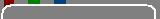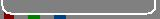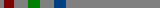Density MatrixMeasurementClifford AlgebraSnuarkBrannenworksThis website is one of five websites founded and maintained by Carl Brannen. Three of these websites are devoted to educating the public in three subjects that I think are important to the foundations of physics, quantum mechanics and especially elementary particle physics for spin-1/2 particles. There are many possible ways of defining the foundations of quantum mechanics. While the broad ideas presented here are not new, the synergies that emerge when they are used in combination is.

## DensityMatrix.com

The purpose of www.DensityMatrix.com is to promote the understanding of the density matrix formulation of quantum mechanics. As quantum mechanics is usually taught, density matrices are derived functions that are mainly useful for modeling mixed states. In this website, I show that the derivation can go both ways, at least for the spin-1/2 fermions. Here we assume that the pure density matrices (pure density matrices which are those which are unchanged by squaring) are fundamental. From them, we derive the spinors. In a certain sense, the density matrix formulation is a return to Von Neumann's program for an algebraic foundation for quantum mechanics. The modification is that we are now connecting the algebra to the geometry of spacetime by way of David Hestenes' geometric calculus or geometric algebra. In that sense the roots of the theory date to the earliest days of quantum mechanics, and the objective of the theory is to achieve Einstein's goal of geometrizing quantum mechanics. This is in distinction to Hestenes' method, which is to geometrize the spinor rather than the density matrix. The primary advantage of choosing the density matrix formalism for geometrization is that it avoids unphysical gauge freedom, a subject of some debate and or confusion.

The usual use of density matrices is well known so my website concentrates on how one gets the spinors from pure density matrices. In doing this, we see that the U(1) gauge symmetry appears as a geometric choice. It is the objective of the rest of these websites to expand this geometrization to the remaining gauge symmetries.

## MeasurementAlgebra.com

The purpose of www.MeasurementAlgebra.com is to promote the understanding of Julian Schwinger's "measurement algebra". The measurement algebra is a particularly elegant method of defining the foundations of quantum mechanics that dates to the 1950s. Schwinger was looking for a way of defining quantum mechanics that was based on the known facts of microscopic measurements of elementary particles. The act of making such a measurement will typically destroy previous knowledge of the particle.

Schwinger then looks at what happens when various experiments are consecutively conducted on an elementary particle (or on a beam of them). For example, if the measurements are of the spin of spin-1/2 particles, then the measuring apparatus is the Stern-Gerlach experiment. If we were to restrict our particles to be only electrons and we only measured spin, then the measurement algebra would represent each particle with the projection operator corresponding to the orientation of the Stern-Gerlach apparatus that gives that spin. But we can be more general than this and imagine an experiment that separates, for example, muons from electrons.

If a measurement is repeated on the same particle, the result of the measurement is unchanged. Schwinger represents this by saying that such a measurement, when squared, gives itself. This is identical to the definition of the pure density matrices -- therefore, we can use Schwinger's measurement algebra to extend the density matrix formulation to more general particles. For example, instead of working with the (single particle) density matrix for an electron, we can consider a single particle density matrix for a lepton, a particle that is either an electron, a muon, a tau, or one of the neutrinos. This is useful in flavor physics.

## CliffordAlgebra.com

The purpose of www.CliffordAlgebra.com is to promote the understanding of Clifford algebra, particularly in its application to the density matrix formulation extended by Schwinger's measurement algebra. Physicists already use two Clifford algebras in the standard model, the Pauli algebra and the Dirac algebra. The subject is usually taught by defining particular representations of these algebras; these are known as the Pauli spin matrices and the Dirac gamma matrices.

The density matrix formulation of quantum mechanics is written in the Pauli or Dirac algebra. In extending this formulation with Schwinger's measurement algebra we need a larger algebra than even the Dirac algebra and this is where Clifford algebra comes in.

The usual way quantum mechanics is presented has the particles represented by vectors while the operators are represented by matrices. The equations one solves are of the form M|m> = m|m>, where M is an operator, and |m> is an eigenvector with eigenvalue m. These are linear equations.

In the density matrix formulation, there is no splitting of the elements into operators and eigenvectors. Instead, all elements are operators, or matrices. The equations one solves are of the form (|m>
But the mathematics of Clifford algebras is sufficiently advanced that it is possible to solve these sorts of equations. While more difficult, it is here that I hope to find the foundations of the elementary particles. To do this, a knowledge of Clifford algebras is necessary.

There are plenty of sources of information on Clifford algebras on the web. A good place to begin is Wikipedia on Clifford Algebra. These sources generally treat the "canonical basis vectors" as fundamental, and define the rest of the algebra in terms of these. This follows the mathematical definition of the algebras. But for the purpose of applying Clifford algebra to Schwinger's measurement algebra, we need an analysis of Clifford algebras from the point of view of particles. That is, we need an analysis that concentrates on the elements of the algebra that satisfy M M = M. These turn out to be related to the elements that square to one.

## Snuark

www.Snuark.com is for my preon theory of elementary particles. It is based on the above observations. To understand it, you will first need to learn the appropriate mathematics and get used to my notation.

## BrannenWorks.com

www.Brannenworks.com was originally started so that I could sell porcelain on the web. This engrossing hobby was driven out by a desire to better understand the foundations of physics. Since early 2003, my website has been mostly about physics. I will continue to keep my papers there, along with various human interest items, Java applets, etc.

## How Am I doing???

It is very difficult to get much attention in the elementary physics community. In particular, trying to get a busy physicist to read a paper that will require learning new mathematical techniques is essentially impossible for an amateur. The only paper I've written that has received much attention was one that had all the "adult" mathematics removed from it. This paper (MASSES2.pdf) is on the masses of the leptons, particularly the neutrinos. It provided an extension of Yoshio Koide's famous empirical formula (hep-ph/0505220) for the masses of the charged leptons.

The way I got the paper read was by carefully eliminating all the things that would prevent a professional physicist from reading it. First I got rid of all the references to alternative foundations for physics. Then I got rid of any new mathematics that anyone would find inconvenient. Then I boiled the result down to just a few very simple equations. This got through, and as a result my formula for the neutrino masses appears in (hep-ph/0605074) . If you are interested in finding other places my formula is mentioned on the web, a useful google search is (koide+brannen+neutrinos)

At the same time that I am typing up the above three informational websites, I am also working on my next paper. This one will bring back the adult mathematics into the lepton mass formulas, and is intended to derive the standard model from a very small number of very simple principles. In the present environment, with very few people who understand the mathematics involved, the paper is pointless. As far as the vast majority of possible readers, it will consist of some empirical formulas, and a bunch of confusing symbols. Thus this paper is a joint effort with these websites.

Carl Brannen

Upon Julia's Clothes

Whenas in silks my Julia goes,
Then, then, methinks, how sweetly flows
That liquefaction of her clothes.

Next, when I cast mine eyes, and see
That brave vibration each way free,
Oh, how that glittering taketh me!

Robert Herrick, 1591-1674

 Web template by : Steve's website templates Template #29